# PSAT Math : Geometry

## Example Questions

### Example Question #651 : Geometry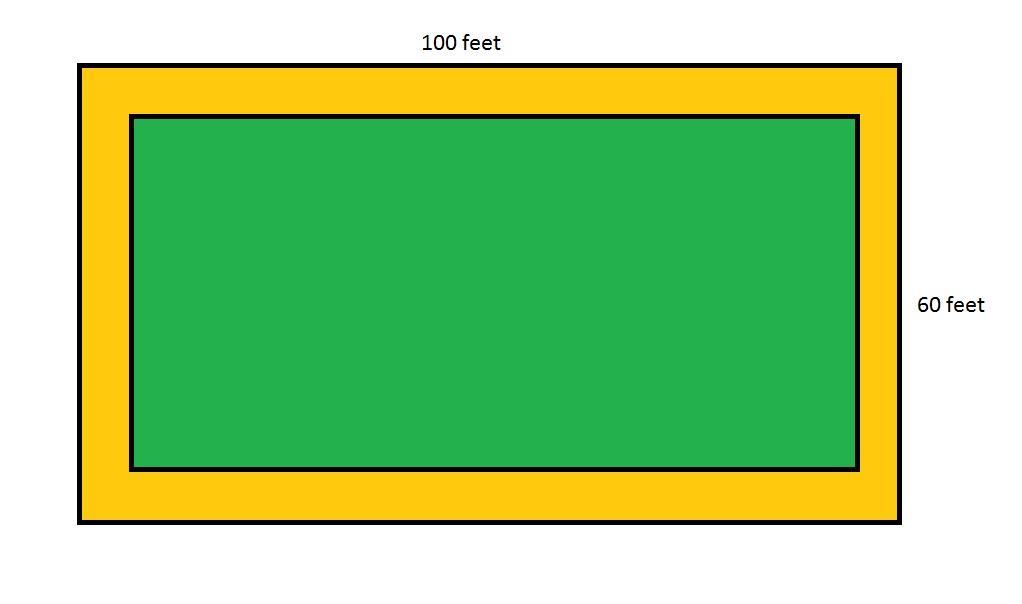Note:  Figure NOT drawn to scale

Refer to the above figure, which shows a rectangular garden (in green) surrounded by a dirt path (in orange) eight feet wide throughout. What is the area of that dirt path?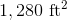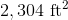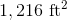The correct area is not given among the other responses.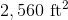Explanation:

The dirt path can be seen as the region between two rectangles. The outer rectangle has length and width 100 feet and 60 feet, respectively, so its area is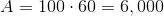square feet.

The inner rectangle has length and width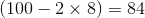feet and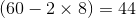feet, respectively, so its area is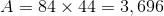square feet.

The area of the path is the difference of the two: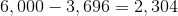square feet.

### Example Question #652 : Geometry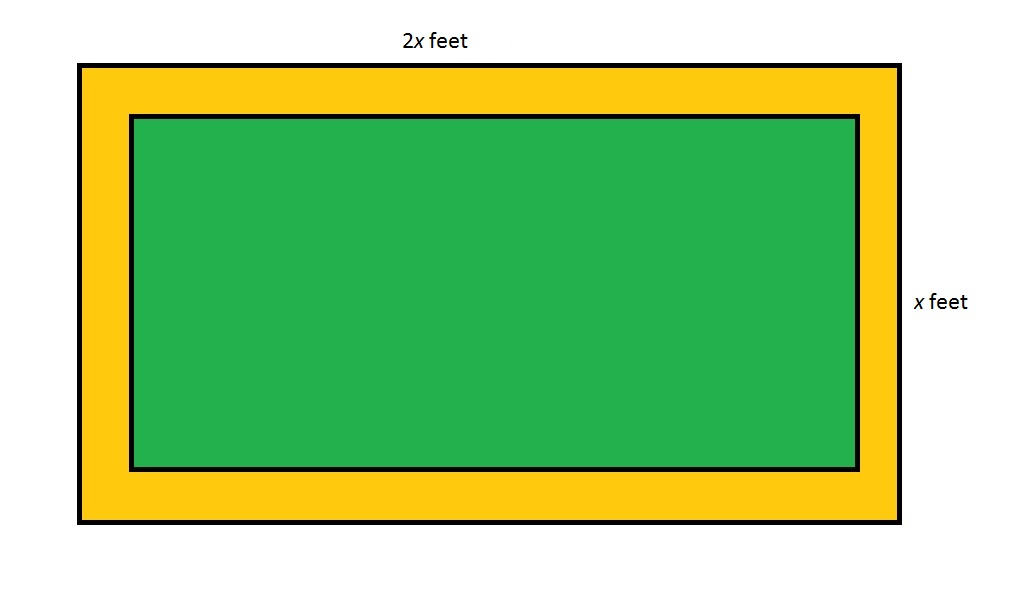Refer to the above figure, which shows a rectangular garden (in green) surrounded by a dirt path (in orange). The dirt path is seven feet wide throughout. Which of the following polynomials gives the area of the dirt path in square feet?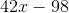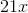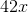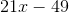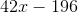Explanation:

The area of the dirt path is the difference between the areas of the outer and inner rectangles.

The outer rectangle has area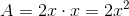The area of the inner rectangle can be found as follows:

The length of the garden is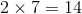feet less than that of the entire lot, or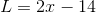;

The width of the garden isless than that of the entire lot, or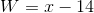;

The area of the garden is their product: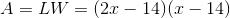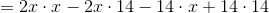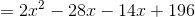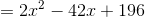Now, subtract the areas: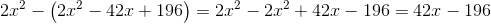### Example Question #7 : How To Find The Area Of A Rectangle

Two circles of a radius of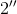each sit inside a square with a side length of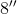.  If the circles do not overlap, what is the area outside of the circles, but within the square?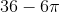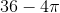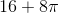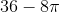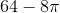Explanation:

The area of a square =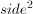The area of a circle is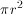Area  = Area of Square2(Area of Circle) =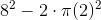### Example Question #653 : Geometry

If the area Rectangle A islarger than Rectangle B and the sides of Rectangle A areand, what is the area of Rectangle B?Explanation: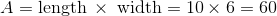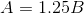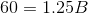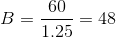### Example Question #4 : Quadrilaterals

ABCD is a parallelogram. BD = 5. The angles of triangle ABD are all equal. What is the perimeter of the parallelogram?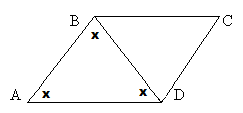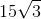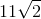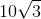Explanation:

If all of the angles in triangle ABD are equal and line BD divides the parallelogram, then all angles in triangle BDC must be equal as well.

We now have two equilateral triangles, so all sides of the triangles will be equal.

All sides therefore equal 5.

5+5+5+5 = 20

### Example Question #6 : How To Find An Angle Of A Line

Two angles are supplementary and have a ratio of 1:4.  What is the size of the smaller angle?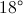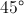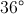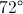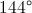Explanation:

Since the angles are supplementary, their sum is 180 degrees.  Because they are in a ratio of 1:4, the following expression could be written: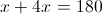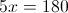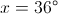### Example Question #7 : How To Find An Angle Of A Line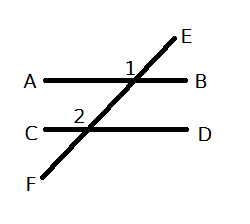AB and CD are two parrellel lines intersected by line EF. If the measure of angle 1 is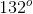, what is the measure of angle 2?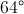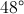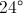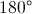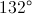Explanation:

The angles are equal. When two parallel lines are intersected by a transversal, the corresponding angles have the same measure.

### Example Question #1 : How To Find The Angle Of Two Lines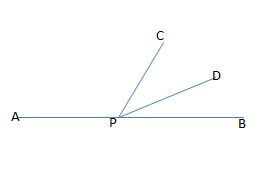Figure not drawn to scale.

In the figure above, APB forms a straight line. If the measure of angle APC is eighty-one degrees larger than the measure of angle DPB, and the measures of angles CPD and DPB are equal, then what is the measure, in degrees, of angle CPB?

114

33

40

66

50

66

Explanation:

Let x equal the measure of angle DPB. Because the measure of angle APC is eighty-one degrees larger than the measure of DPB, we can represent this angle's measure as x + 81. Also, because the measure of angle CPD is equal to the measure of angle DPB, we can represent the measure of CPD as x.

Since APB is a straight line, the sum of the measures of angles DPB, APC, and CPD must all equal 180; therefore, we can write the following equation to find x:

x + (x + 81) + x = 180

Simplify by collecting the x terms.

3x + 81 = 180

Subtract 81 from both sides.

3x = 99

Divide by 3.

x = 33.

This means that the measures of angles DPB and CPD are both equal to 33 degrees. The original question asks us to find the measure of angle CPB, which is equal to the sum of the measures of angles DPB and CPD.

measure of CPB = 33 + 33 = 66.

### Example Question #2 : Geometry

One-half of the measure of the supplement of angle ABC is equal to the twice the measure of angle ABC. What is the measure, in degrees, of the complement of angle ABC?

90

36

72

18

54

54

Explanation:

Let x equal the measure of angle ABC, let y equal the measure of the supplement of angle ABC, and let z equal the measure of the complement of angle ABC.

Because x and y are supplements, the sum of their measures must equal 180. In other words, x + y = 180.

We are told that one-half of the measure of the supplement is equal to twice the measure of ABC. We could write this equation as follows:

(1/2)y = 2x.

Because x + y = 180, we can solve for y in terms of x by subtracting x from both sides. In other words, y = 180 – x. Next, we can substitute this value into the equation (1/2)y = 2x and then solve for x.

(1/2)(180-x) = 2x.

Multiply both sides by 2 to get rid of the fraction.

(180 – x) = 4x.

180 = 5x.

Divide both sides by 5.

x = 36.

The measure of angle ABC is 36 degrees. However, the original question asks us to find the measure of the complement of ABC, which we denoted previously as z. Because the sum of the measure of an angle and the measure of its complement equals 90, we can write the following equation:

x + z = 90.

Now, we can substitute 36 as the value of x and then solve for z.

36 + z = 90.

Subtract 36 from both sides.

z = 54.

### Example Question #3 : Geometry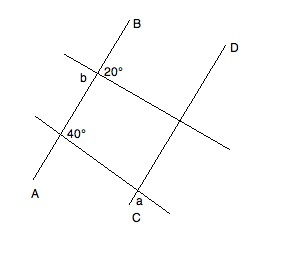In the diagram, AB || CD. What is the value of a+b?

160°

140°

80°

60°

160°

Explanation:

Refer to the following diagram while reading the explanation: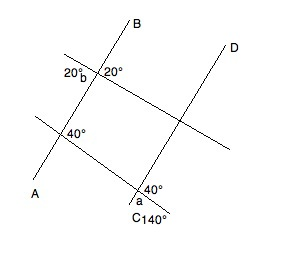We know that angle b has to be equal to its vertical angle (the angle directly "across" the intersection).  Therefore, it is 20°.

Furthermore, given the properties of parallel lines, we know that the supplementary angle to a must be 40°.  Based on the rule for supplements, we know that a + 40° = 180°.  Solving for a, we get a = 140°.

Therefore, a + b = 140° + 20° = 160°

### All PSAT Math Resources